American Journal of Software Engineering and Applications
Volume 6, Issue 1, February 2017, Pages: 1-4

Computation of 10 Knife Edge Diffraction Loss Using Epstein-Peterson Method

Wali Samuel1, Trust Christopher Oguichen2, Steve Worgu2

1Department of Electrical/Electronic and Computer Engineering, University of Uyo, Uyo, Nigeria

2Department of Electrical/Computer Engineering, Port Harcourt Polytechnic, Rumuola, Port Harcourt, Nigeria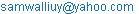(W. Samuel)

Wali Samuel, Trust Christopher Oguichen, Steve Worgu. Computation of 10 Knife Edge Diffraction Loss Using Epstein-Peterson Method. American Journal of Software Engineering and Applications. Vol. 6, No. 1, 2017, pp. 1-4. doi: 10.11648/j.ajsea.20170601.11

Received: January 3, 2017; Accepted: January 10, 2017; Published: February 3, 2017

Abstract: In this paper, application of Epstein-Peterson method in the computation of a ten (10) multiple knife edge diffraction loss is presented for a 1 GHz microwave link. In the computation, each of the ten obstructions gave rise to a virtual hop which resulted in a knife edge diffraction loss. What is peculiar to the Epstein-Peterson method is how the virtual hops are identified or defined. The overall diffraction loss, according to the Epstein-Peterson method is the sum of the diffraction loss computed for each of the ten virtual hops. In the results, the highest LOS clearance height of 5.727273 m occurred in virtual hop 5 whereas the highest diffraction parameter of 0.333333 and the highest virtual hop diffraction loss of 8.908754dB occurred in virtual hop1. The lowest LOS clearance height of 0.4 m, the lowest diffraction parameter 0.029814 and the lowest virtual hop diffraction loss, 6.290874 dB occurred in virtual hop 9. In all, the overall effective diffraction loss for the 10 knife edge obstructions as computed by the Epstein-Peterson is 69.93384 dB.

Keywords: Multiple Knife Edge, Diffraction Loss, Diffraction Parameter, Line of Sight, Clearance Height, Virtual Hop, Epstein-Peterson Method

Contents

1. Introduction

In multiple knife edge diffraction loss calculation, two or more knife egde obstructions are considered where the height of each of the obstructions extends above the line of site (LOS) [1-5]. Analysis of such multiple knife edge diffraction can be done in several ways. However, in most cases, the analysis is limited to a maximum of three obstructions because of the increasing complexity of the analysis of more than three multiple knife edge obstructions [6-9]. In this paper, an approach for the determination of ten (10) multiple knife edge diffraction loss using Epstein-Peterson multiple knife edge diffraction loss method is presented [10-14]. Sample 10 knife edge obstructions are used to demonstrate the applicability of the approach for a 1GHz microwave signal.

2 Epstein-Peterson Multiple Knife Edge Diffraction Loss Method

Figure 1 shows a three knife edge obstructions used to present the basic principles of the Epstein-Peterson multiple knife edge diffraction loss method. In figure 1 each of the obstructions that blocks the line of sight consitutes an edge that will cause diffraction loss and also introduces a virtual hop in the link. Each virtual hop has an edge that causes diffraction.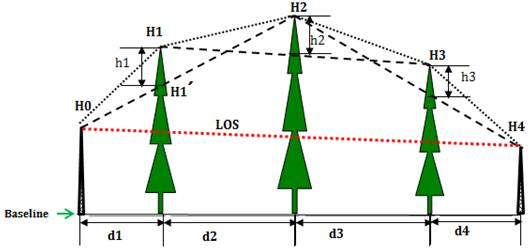Figure 1. None Line –Of Sight Link With Three Knife Edge Obstructions.

In figure 1, there are three virtual hops, namely;

i.    Hop1: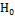-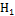-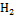with H1 as the diffraction edge

ii.   Hop2:--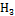with H2 as the diffraction edge

iii.   Hop3:--with H3 as the diffraction edge

Consider hop 1 in figure 1, the LOS clearance height,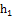is given as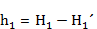(1)

Where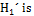the hop 1 line of sight (To) height at a distance of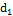from.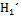is given by similar traingle as ;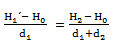(2)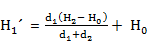(3)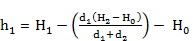(4)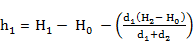(5)

Similarly ,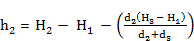(6)

Generally, in the Epstein-Peterson multiple knife edge diffraction loss method, for any given hop j, the clearance height to its LOS is given as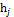where;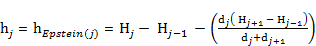(7)

The knife-edge diffraction parameter for any hop j is given as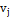where;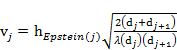(8)

According to ITU (Rec 526-13, 2011) the knife-edge diffraction loss, A for any given diffraction parameter, v is given as [16,17];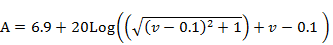(9)

where A is in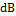Then, in respect of knife-edge diffraction loss for any hop j with diffraction parameter,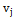, the knife-edge diffraction loss is denoted as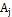, where ITU approximation model foris give as;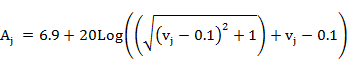(10)

whereis inAccording to the Epstein-Peterson multiple diffraction loss method, the effective diffraction loss for all the m hops is given as;

A =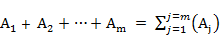(11)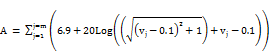(12)

3. Case Study: 10 Knife Edge Diffraction Loss Computation

The case study is a 10 knife edge obstructions as shown in figure 2. The 10 knife edge obstruction have heights H1, H2,…,H10 while H0 and H11 are the transmitter and receiver heights respectively. Also, the distance of obstruction (i+1) from obstruction (i) is d(i+1) where i= 0,1,2,…,10. Again, for the transmitter i=0 and for the receiver i=11. Table 1 gives the height, H(i) and the distance d(i) between adjacent obstructions for the 10 knife edge obstructions along with the transmitter and the receiver. The dataset in Table 1 and Figure 2 are used to present numerical computations of the 10 knife edge diffraction loss using the Epstein-Peterson method.

Table 1. The Height of the Ten (10) Knife Edge Obstructions and the distance between adjacent obstructions.

 Distance(km) Between Adjacent Obstructions Knife Edge Obstruction Height (Transmitter) H0 10 d1 1 H1 18 d2 2 H2 24 d3 3 H3 30 d4 4 H4 36 d5 5 H5 42 d6 6 H6 45 d7 5 H7 37 d8 4 H8 28 d9 3 H9 20 d10 2 H10 14 d11 1 (Receiver) H11 10 d 36 F =1GHz λ = 0.3

Table 2. The LOS Clearance Height, h(j), The Diffraction Parameter, V(j) and The Diffraction Loss, A(j) For The 10 Virtual Hops As Computed By Epstein-Peterson Method.

 j H(j-1) H(j) H(j+1) d(j) d(j+1) h(j) V(j) A(j) 1 10 18 24 1 2 3.333333 0.333333 8.908754 2 18 24 30 2 3 1.2 0.089443 6.808302 3 24 30 36 3 4 0.857143 0.053452 6.495837 4 30 36 42 4 5 0.666667 0.036515 6.348945 5 36 42 45 5 6 1.909091 0.094388 6.851255 6 42 45 37 6 5 5.727273 0.283164 8.482178 7 45 37 28 5 4 1.444444 0.079115 6.718613 8 37 28 20 4 3 0.714286 0.044544 6.418558 9 28 20 14 3 2 0.4 0.029814 6.290874 10 20 14 10 2 1 0.666667 0.066667 6.610524 f 1GHz λ 0.3 Total 69.93384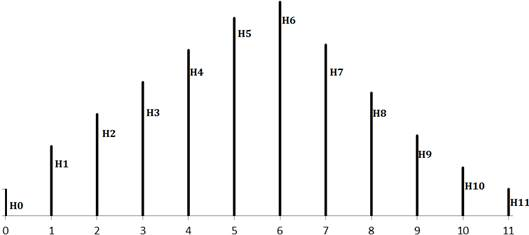Figure 2. Ten (10) Knife Edge Obstructions.

Generally, in multiple knife edge diffraction loss methodologies, each knife edge constitutes a virtual hop with two adjacent knife edge obstructions, or with the transmitter and a knife edge obstruction or with a knife edge obstruction and with the receiver. In this study, the 10 knife edge obstructions gave rise to 10 virtual hops. In Table 2, the results of the LOS clearance height, h(j), the diffraction parameter, V(j) and the diffraction loss, A(j) computed for the 10 virtual hops using the Epstein-Peterson method are presented. The highest LOS clearance height h(j) =5.727273 m occurred in virtual hop j =5 as shown in Table 2 and figure 3. However, the highest diffraction parameter, V(j) =0.333333 is obtained in virtual hop j =1, as shown in Table 2 and figure 3 and figure 4. The lowest LOS clearance height h (j) =0.4 m occurred in virtual hop j = 9. Also, the lowest diffraction parameter, V(j) =0.029814 occurred in virtual hop j = 9.

In Table 2 and figure 4 the lowest virtual hop diffraction loss, A(j) =6.290874 dB occurred in virtual hop j = 9 whereas, the highest virtual hop diffraction loss, A(j) =8.908754dB occurred in virtual hop j =1. In all, the overall effective diffraction loss for the 10 knife edge obstructions as computed by the Epstein-Peterson method is 69.93384 dB.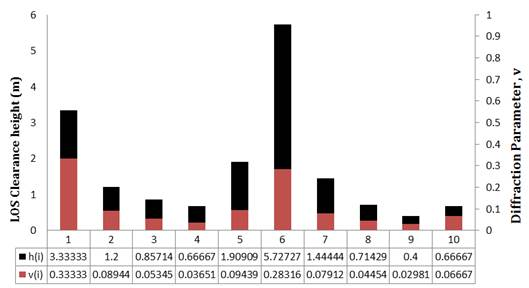Figure 3. The LOS Clearance Heights, h(j) and Diffraction Parameter, V(j) For The 10 Virtual Hops Of The 10 Knife Edge Obstructions.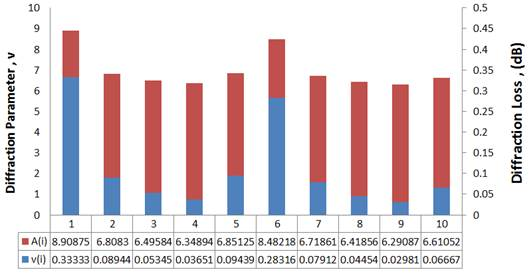Figure 4. The Diffraction Parameter, V(j) and the Diffraction Loss, A(j) For The 10 Virtual Hops Of The 10 Knife Edge Obstructions.

4. Conclusion

Ten multiple knife edge diffraction loss computation with Epstein-Peterson method is presented. The study is conducted for a 1 GHz microwave line link. In the computation, each of the ten obstructions gave rise to a virtual hop which resulted in a knife edge diffraction loss. The overall diffraction loss, according to the Epstein-Peterson method is the sum of the diffraction loss computed for each of the ten virtual hops. What is peculiar to the Epstein-Peterson method is how the virtual hops are identified or defined.

References

1. Bibb, D. A., Dang, J., Yun, Z., & Iskander, M. F. (2014, July). Computational accuracy and speed of some knife-edge diffraction models. In 2014 IEEE Antennas and Propagation Society International Symposium (APSURSI) (pp. 705-706). IEEE.
2. Lazaridis, P. I., Kasampalis, S., Zaharis, Z. D., Cosmas, J. P., Paunovska, L., & Glover, I. (2015, May). Longley-Rice model precision in case of multiple diffracting obstacles. In URSI Atlantic Conference, Canary Islands.
3. Zeng, D., Lu, G., & Zhang, R. (2015, October). Radiowave propagation loss measurement of different situated Knife-Edge problems and comparison with PO computing. In Microwave, Antenna, Propagation, and EMC Technologies (MAPE), 2015 IEEE 6th International Symposium on(pp. 40-43). IEEE.
4. Han, T., Kuang, Z., Wang, H., & Li, X. (2015, April). Study on the multiple diffraction for UWB signals under NLOS environment in WSNs. In 2015 International Conference on Intelligent Systems Research and Mechatronics Engineering. Atlantis Press.
5. Topcu, S., Goktas, P., Karasan, E., & Altintas, A. (2015, September). A new approach to diffraction modelling for line-of-sight (LOS) paths. In Antennas and Propagation in Wireless Communications (APWC), 2015 IEEE-APS Topical Conference on(pp. 696-699). IEEE.
6. Baldassaro, P. M. (2001). RF and GIS: Field Strength Prediction for Frequencies between 900 MHz and 28 GHz.
7. Boban, M., Vinhoza, T. T., Ferreira, M., Barros, J., & Tonguz, O. K. (2011). Impact of vehicles as obstacles in vehicular ad hoc networks. IEEE journal on selected areas in communications, 29 (1), 15-28.
8. Phillips, C., Sicker, D., & Grunwald, D. (2013). A survey of wireless path loss prediction and coverage mapping methods. IEEE Communications Surveys & Tutorials, 15 (1), 255-270.
9. Östlin, E. (2009). On Radio Wave Propagation Measurements and Modelling for Cellular Mobile Radio Networks.
10. Valtr, P., Pechac, P., & Grabner, M. (2015). Inclusion of Higher Order Diffracted Fields in the Epstein–Peterson Method. IEEE Transactions on Antennas and Propagation, 63 (7), 3240-3244.
11. See, T. S., Qing, X., Liu, W., & Chen, Z. N. (2015). A Wideband Ultra-Thin Differential Loop-Fed Patch Antenna for Head Implants. IEEE Transactions on Antennas and Propagation, 63 (7), 3244-3248.
12. Bibb, D. A., Dang, J., Yun, Z., & Iskander, M. F. (2014, July). Computational accuracy and speed of some knife-edge diffraction models. In 2014 IEEE Antennas and Propagation Society International Symposium (APSURSI)(pp. 705-706). IEEE.
13. Kasampalis, S., Lazaridis, P. I., Zaharis, Z. D., Bizopoulos, A., Paunovska, L., Zettas, S.,... & Cosmas, J. (2015, June). Longley-Rice model prediction inaccuracies in the UHF and VHF TV bands in mountainous terrain. In 2015 IEEE International Symposium on Broadband Multimedia Systems and Broadcasting (pp. 1-5). IEEE.
14. Acar, T., Çalışkan, F., & Aydın, E. (2015, April). Comparison of computer-based propagation models with experimental data collected in an urban area at 1800 MHz. In Wireless and Microwave Technology Conference (WAMICON), 2015 IEEE 16th Annual (pp. 1-6). IEEE.
15. Sizun, H., & de Fornel, P. (2005). Radio wave propagation for telecommunication applications. Heidelberg: Springer.
16. ITU-R P. 526-13, "Propagation by diffraction," November 2013.
17. Rieche, M., Ihlow, A., Heyn, T., Pérez-Fontán, F., & Del Galdo, G. (2015, May). Land mobile satellite propagation characteristics from knife-edge diffraction modeling and hemispheric images. In 2015 9th European Conference on Antennas and Propagation (EuCAP) (pp. 1-4). IEEE.

 Contents 1. 2 3. 4.
Article ToolsAbstractPDF(294K)